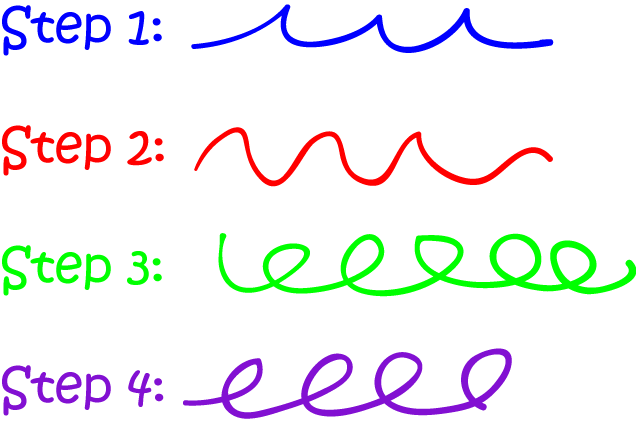# Definition of AlgorithmAn algorithm is a sequence of steps you perform to complete a task like solving a problem or baking a cake.

A recipe is an example of an algorithm.

Some other examples of algorithms are the steps involved in long division, computer programs and the instructions for building a model.

Let's work through the steps of one algorithm for adding three digit numbers (without borrowing or trading):

The algorithm is as follows:

4. combine the three digits from steps 1-3 to give the answer.

Let's use it to add $123$ and $245$:

1. add $3$ and $5$ to give $8$
2. add $20$ and $40$ to give $60$
3. add $100$ and $200$ to give $300$
4. combining these three numbers together gives the answer of $368$.

### Description

The aim of this dictionary is to provide definitions to common mathematical terms. Students learn a new math skill every week at school, sometimes just before they start a new skill, if they want to look at what a specific term means, this is where this dictionary will become handy and a go-to guide for a student.

None

### Audience

Year 1 to Year 12 students

### Learning Objectives

Learn common math terms starting with letter A

Author: Subject Coach
You must be logged in as Student to ask a Question.